## Resolution to Lifestyle

 /* styles */ No Matter The Day - It Will Be Music2YourFeet! -Denise Bryant, Founder
 table div table+table+table+table+table+table div table{width:100%;padding:0}table div table+table+table+table+table+table div table img{width:96.23%;padding:0;float:none}table div table+table+table+table+table+table div table td{width:100%;padding:0 1.88% 18px}/* styles *//* styles */
 /* styles */ National Nutrition Month® is a nutrition education and information campaign created annually in March by the Academy of Nutrition and Dietetics. The campaign focuses attention on the importance of making informed food choices and developing sound eating and physical activity habits. Below are 20 Tips - from the 2020 Eat Right Bite by Bite Campaign. Here's to your Health in 2020! Denise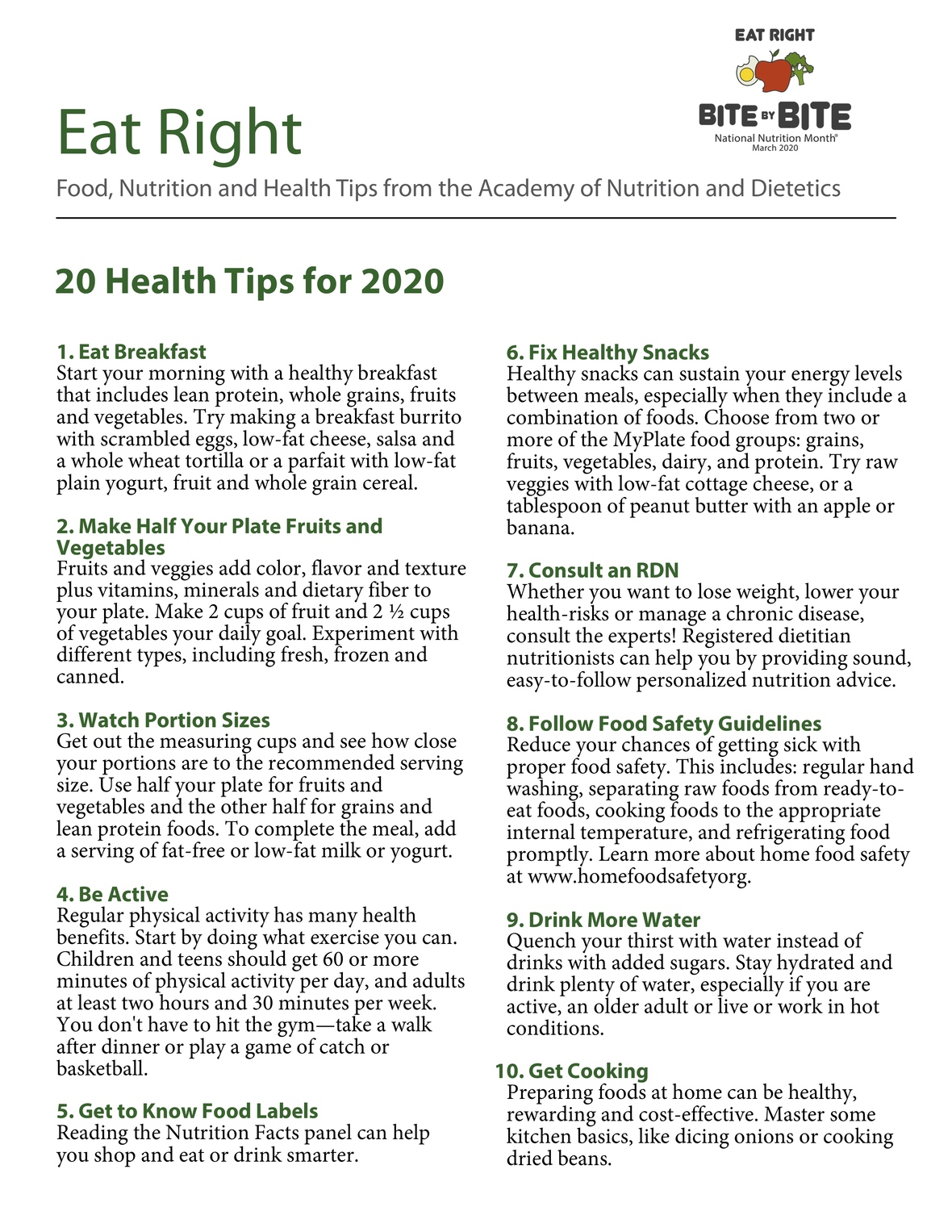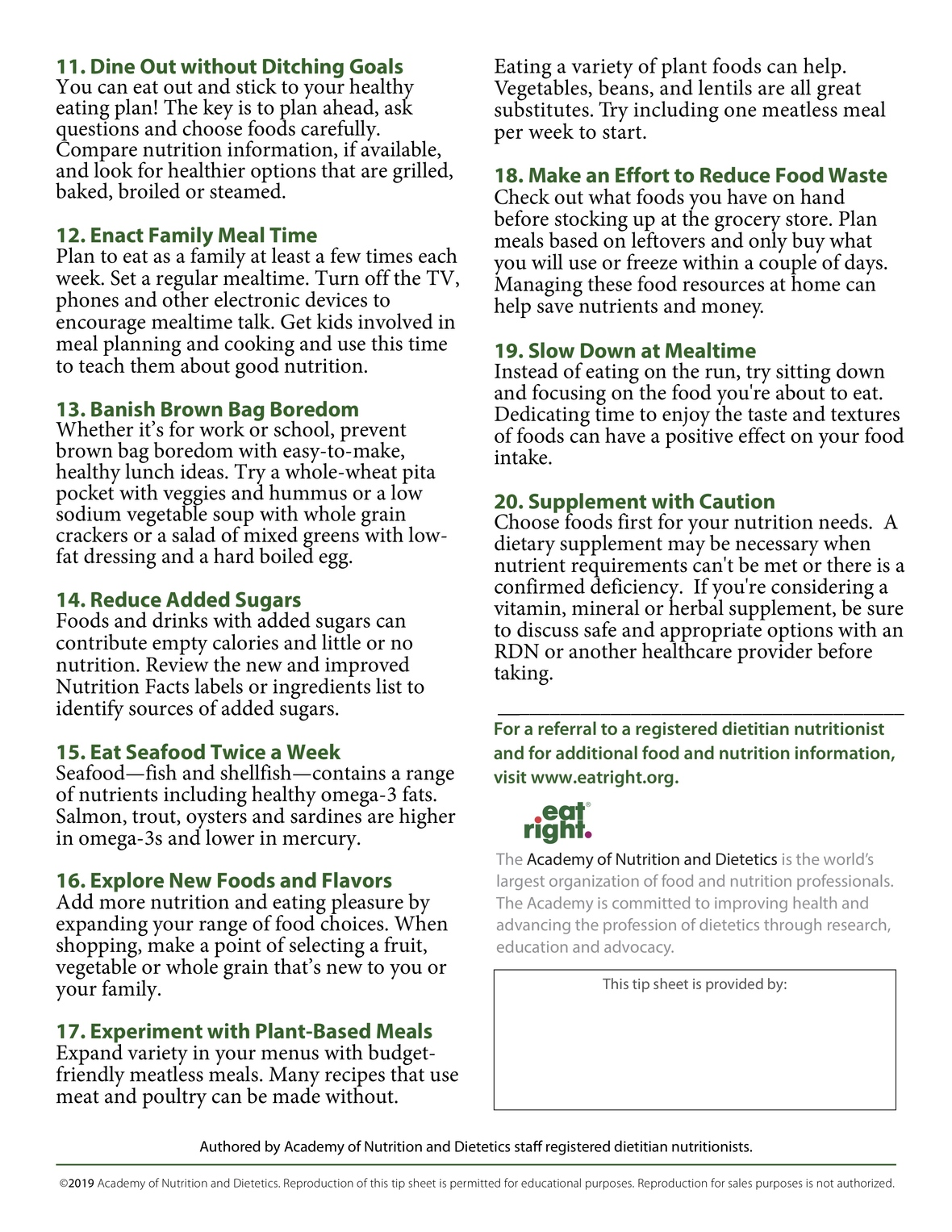table div table+table+table+table+table+table+table+table+table+table+table+table div table{width:100%;padding:0}table div table+table+table+table+table+table+table+table+table+table+table+table div table img{width:96.23%;padding:0;float:none}table div table+table+table+table+table+table+table+table+table+table+table+table div table td{width:100%;padding:0 1.88% 18px}/* styles */## Denise's Corner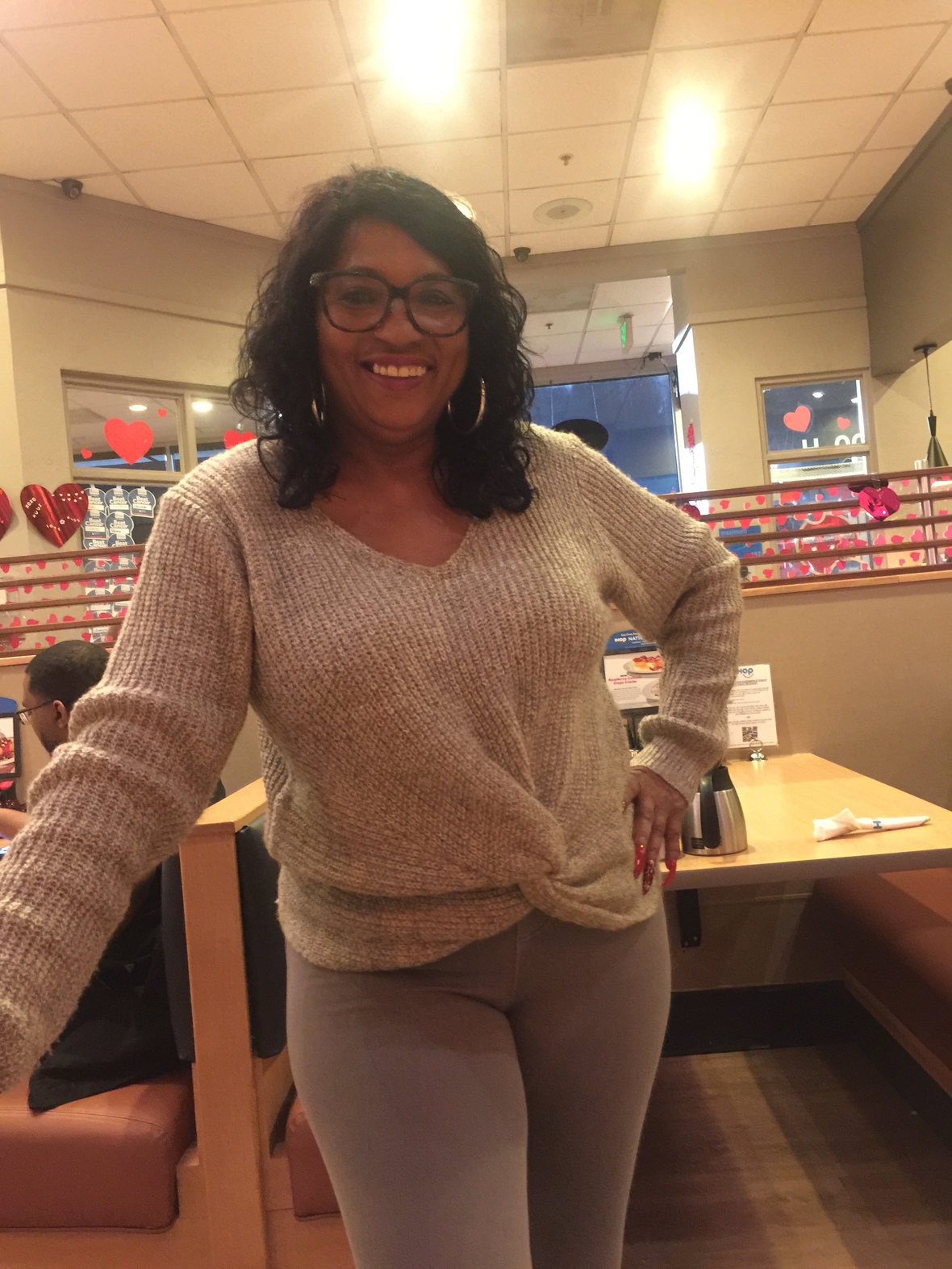We would love to spotlight your event or an individual in the community.

We will feature someone or an event(s) in the next edition of Denise's corner.

Donations not required but appreciated.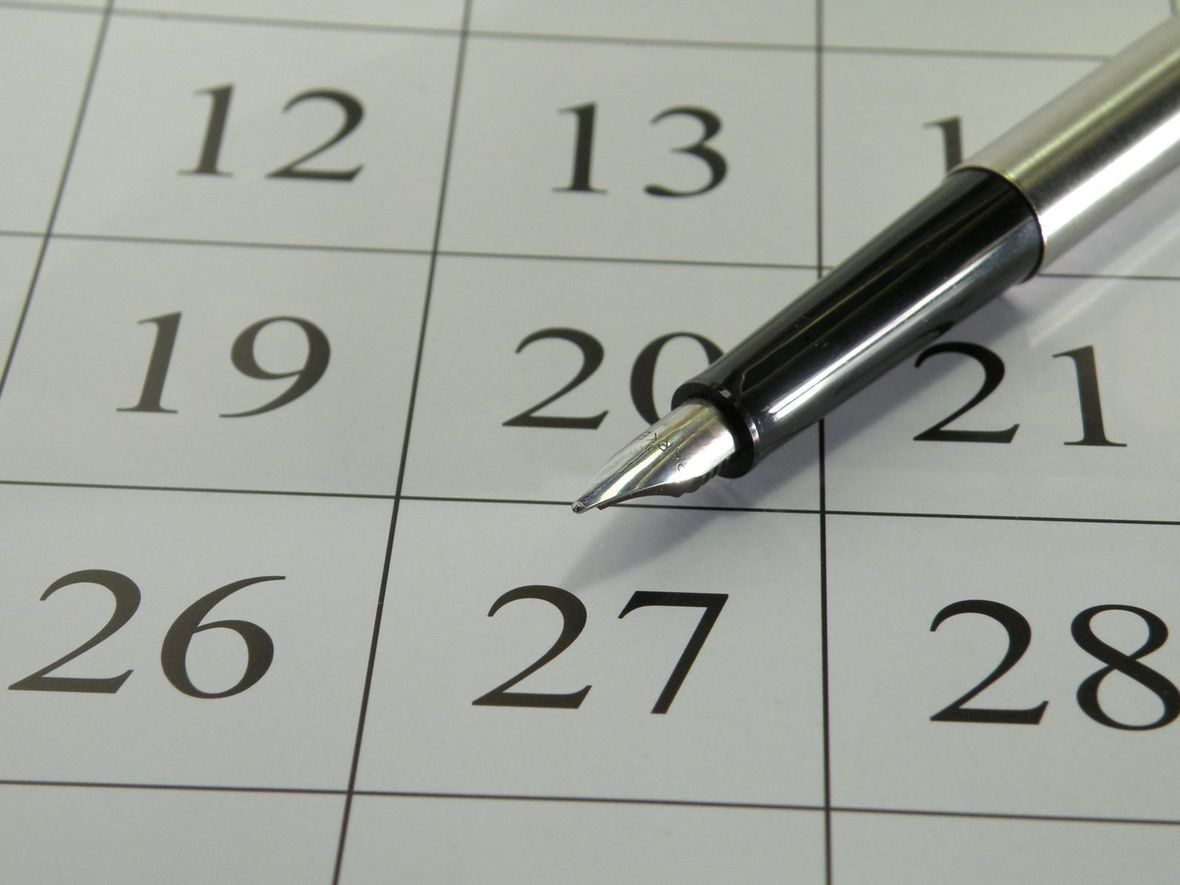# Music2yourfeet 7th Annual Holiday Party

 table div table+table+table+table+table+table+table+table+table+table+table+table+table+table+table+table div table{width:100%;padding:0}table div table+table+table+table+table+table+table+table+table+table+table+table+table+table+table+table div table img{width:96.23%;padding:0;float:none}table div table+table+table+table+table+table+table+table+table+table+table+table+table+table+table+table div table td{width:100%;padding:0 1.88% 18px}/* styles */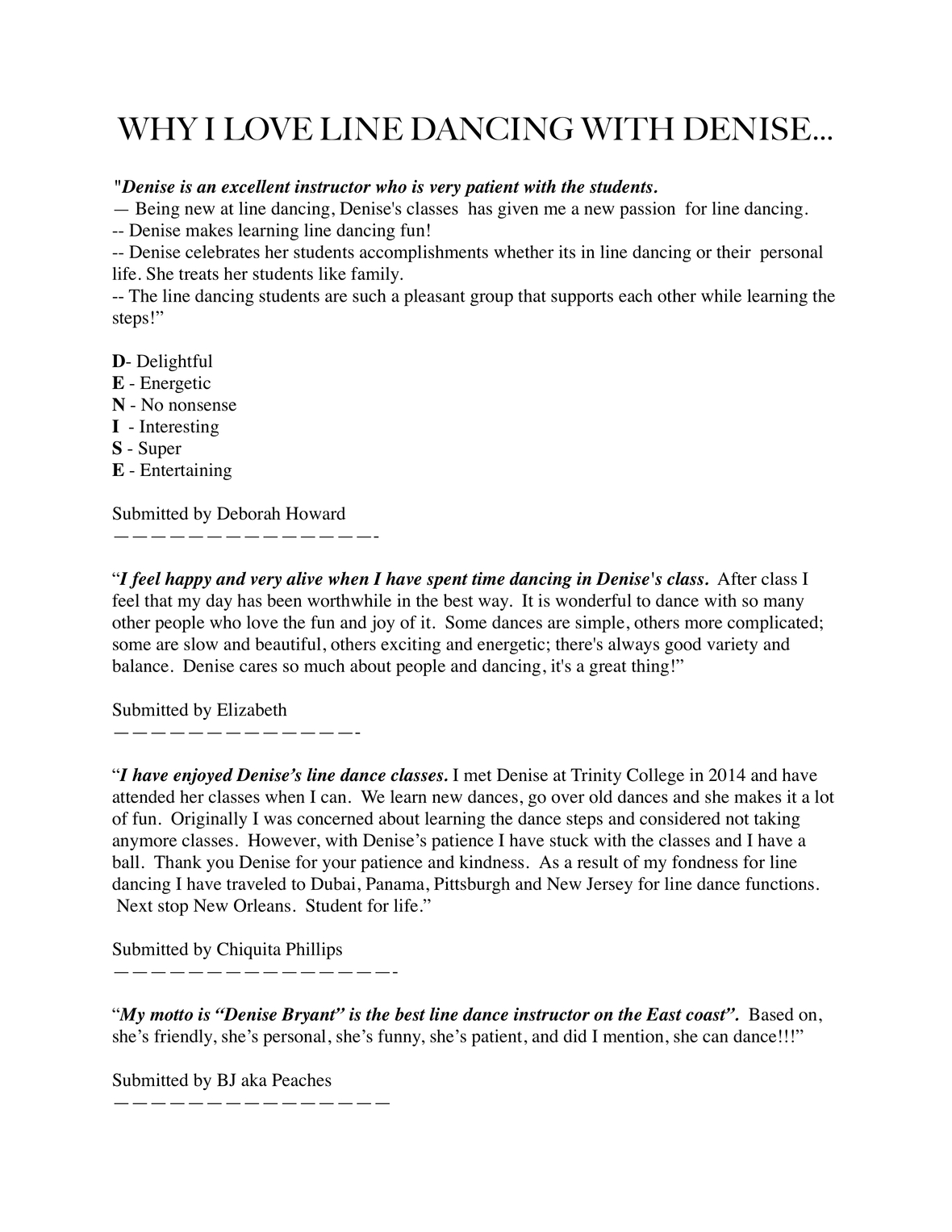table div table+table+table+table+table+table+table+table+table+table+table+table+table+table+table+table+table+table div table{width:100%;padding:0}table div table+table+table+table+table+table+table+table+table+table+table+table+table+table+table+table+table+table div table img{width:96.23%;padding:0;float:none}table div table+table+table+table+table+table+table+table+table+table+table+table+table+table+table+table+table+table div table td{width:100%;padding:0 1.88% 18px}/* styles */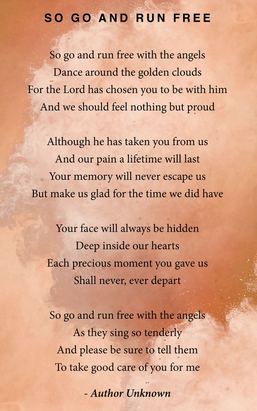# Rest in Peace Lloyd Franklin

You will truly be missed by all.

 table div table+table+table+table+table+table+table+table+table+table+table+table+table+table+table+table+table+table+table+table div table{width:100%;padding:0}table div table+table+table+table+table+table+table+table+table+table+table+table+table+table+table+table+table+table+table+table div table img{width:96.23%;padding:0;float:none}table div table+table+table+table+table+table+table+table+table+table+table+table+table+table+table+table+table+table+table+table div table td{width:100%;padding:0 1.88% 18px}/* styles */table div table+table+table+table+table+table+table+table+table+table+table+table+table+table+table+table+table+table+table+table+table+table div table{width:100%;padding:0}table div table+table+table+table+table+table+table+table+table+table+table+table+table+table+table+table+table+table+table+table+table+table div table img{width:96.23%;padding:0;float:none}table div table+table+table+table+table+table+table+table+table+table+table+table+table+table+table+table+table+table+table+table+table+table div table td{width:100%;padding:0 1.88% 18px}/* styles */## In the Spotlight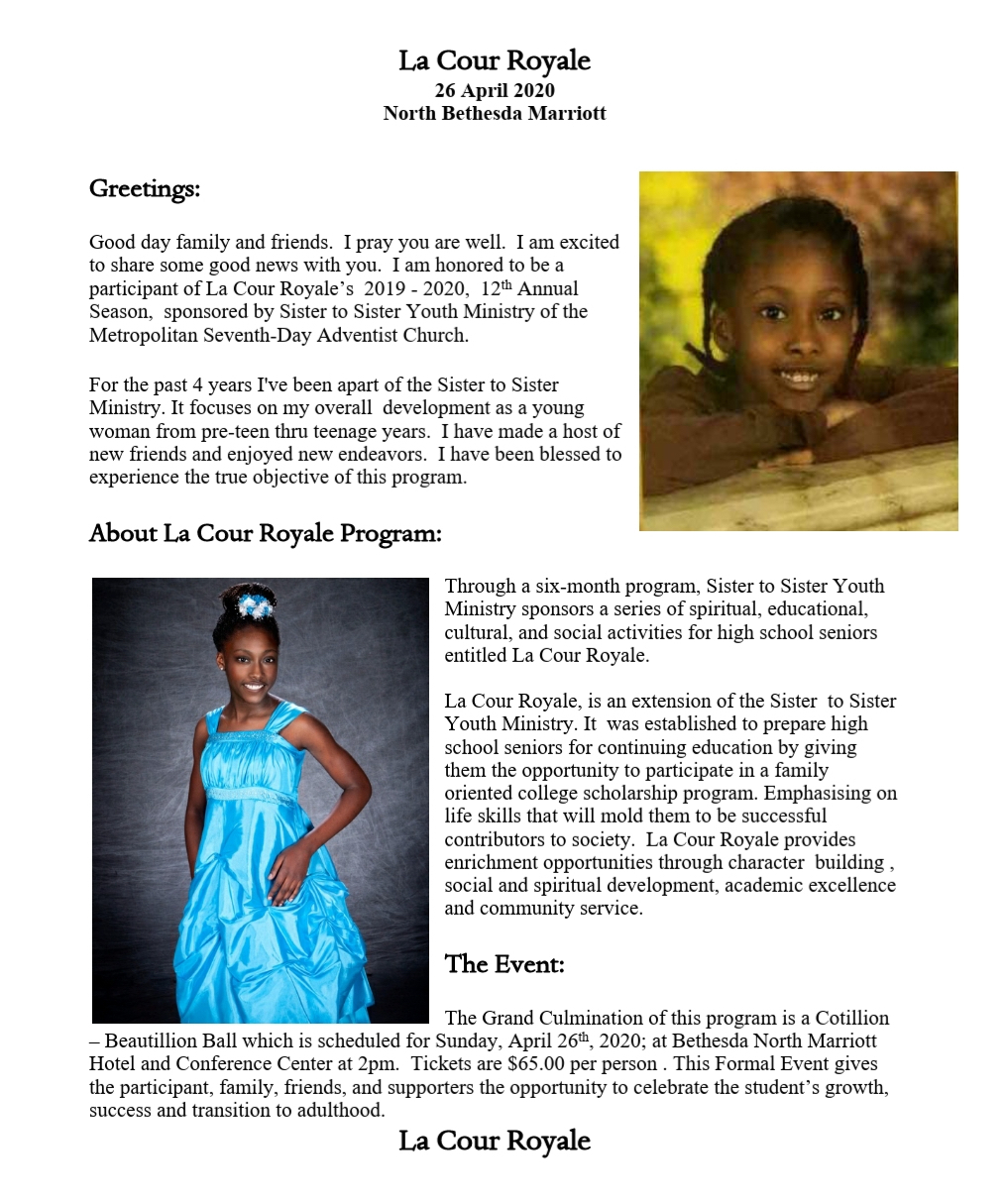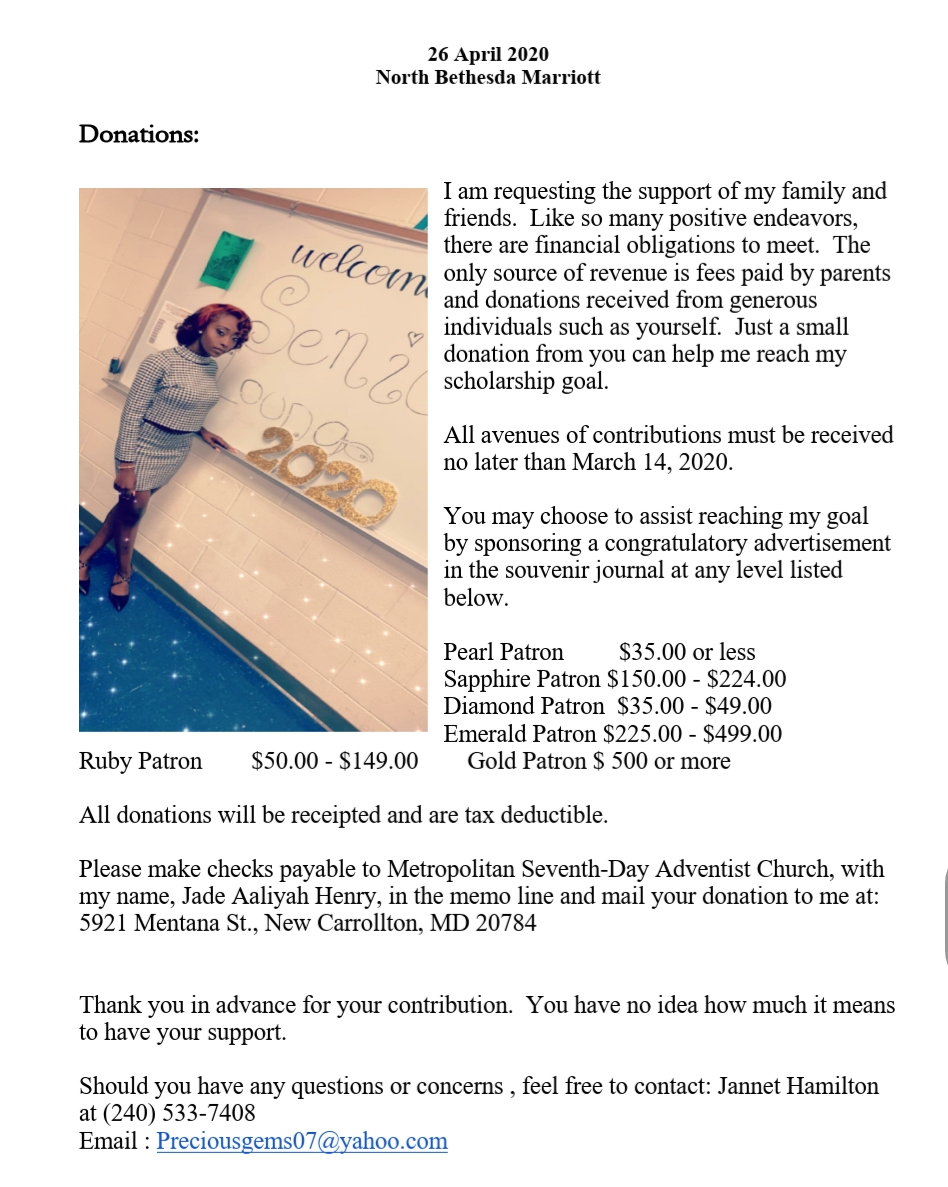table div table+table+table+table+table+table+table+table+table+table+table+table+table+table+table+table+table+table+table+table+table+table+table+table+table+table div table{width:100%;padding:0}table div table+table+table+table+table+table+table+table+table+table+table+table+table+table+table+table+table+table+table+table+table+table+table+table+table+table div table img{width:96.23%;padding:0;float:none}table div table+table+table+table+table+table+table+table+table+table+table+table+table+table+table+table+table+table+table+table+table+table+table+table+table+table div table td{width:100%;padding:0 1.88% 18px}/* styles */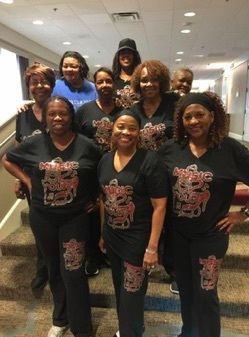Music2YourFeet Workout Suit (2 Piece)

\$40 for set, separate \$20 each,
come in black and gray only, stretch material...size from small to 2x pants and 3X shirt, may use Cash App \$seafo1 to pay, or cash, sorry no paypal and no checks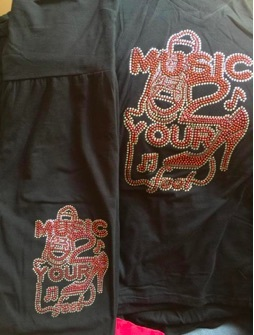Music2YourFeetWorkout Suit (pant and t-shirt)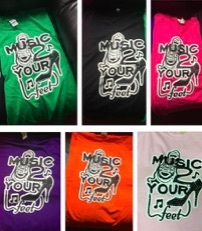Music2YourFeet T-Shirt

\$15 each, may use Cash App \$seafo1 to pay, or cash, sorry no paypal and no checks

Please indicate the T-shirt color when ordering.
Colors available:
Green, Black, Hot Pink, Purple, Orange and Light Pink,

 table div table+table+table+table+table+table+table+table+table+table+table+table+table+table+table+table+table+table+table+table+table+table+table+table+table+table+table+table+table+table+table div table{width:100%;padding:0}table div table+table+table+table+table+table+table+table+table+table+table+table+table+table+table+table+table+table+table+table+table+table+table+table+table+table+table+table+table+table+table div table img{width:96.23%;padding:0;float:none}table div table+table+table+table+table+table+table+table+table+table+table+table+table+table+table+table+table+table+table+table+table+table+table+table+table+table+table+table+table+table+table div table td{width:100%;padding:0 1.88% 18px}/* styles */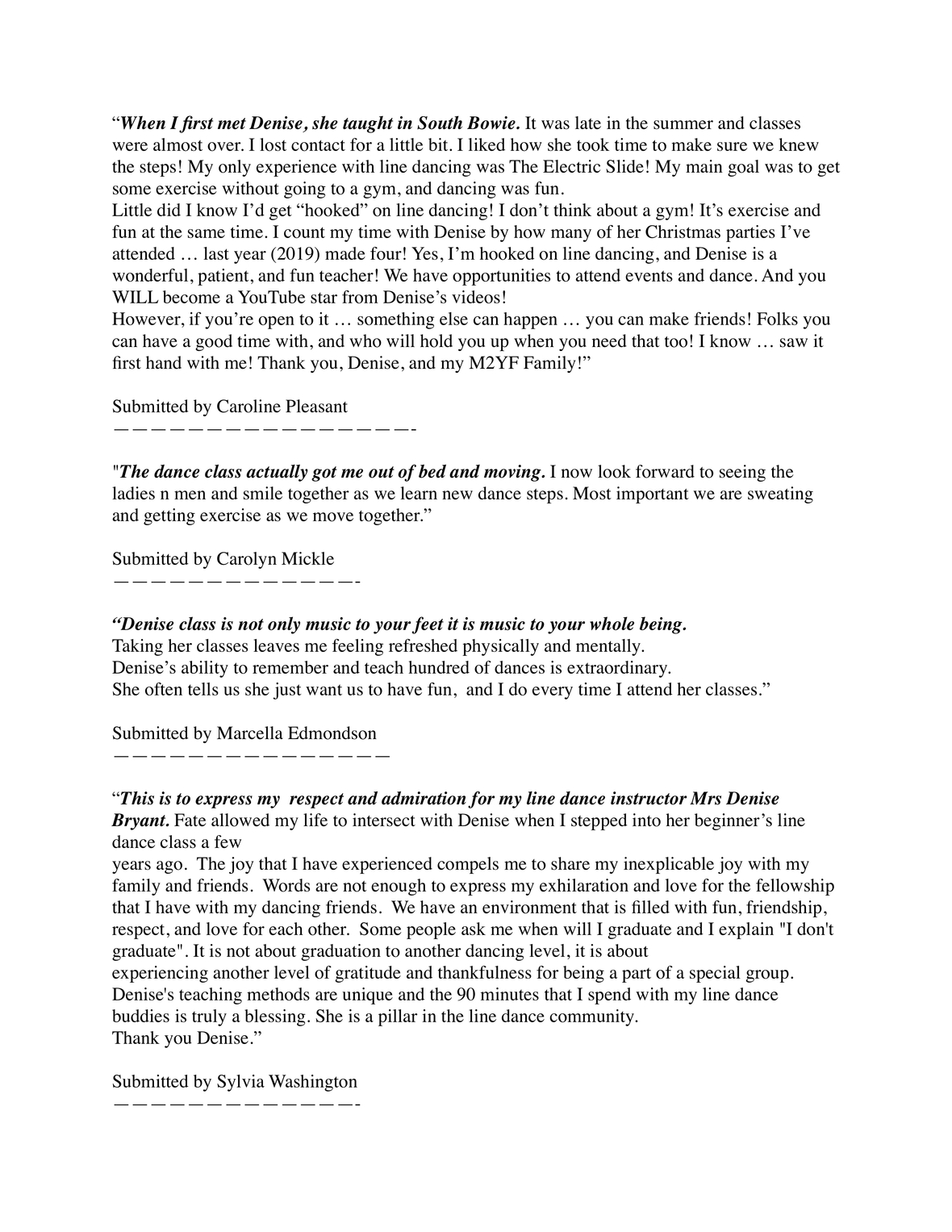## Special Birthday shout outs to my family and friends....

 /* styles */ A very Special Happy 2nd Birthday to (TTB), Maliyah, (Trash Talking Baby), Nephew, Sean Jackson, Happy 50th, Happy Birthday, Auntie Cheryl Brewer,Very Special Birthday to my partner in crime, Ann Douglas, and a very Special Happy Birthday to my Son-In-Law Brian McNeil, Taryn Savoy, my brothers Richard Leroy Kidwell, (Leroy), and Richard C. Kidwell, Jr. (Rick) aka (Rack), Harold Wood (Wendy), Keith Richardson, a very special Happy Birthday to Linda Fung, aka (LD), Cindy Gray, and Delores Herbert. Happy Birthday to all the March babies!table div table+table+table+table+table+table+table+table+table+table+table+table+table+table+table+table+table+table+table+table+table+table+table+table+table+table+table+table+table+table+table+table+table+table+table+table+table div table{width:100%;padding:0}table div table+table+table+table+table+table+table+table+table+table+table+table+table+table+table+table+table+table+table+table+table+table+table+table+table+table+table+table+table+table+table+table+table+table+table+table+table div table img{width:96.23%;padding:0;float:none}table div table+table+table+table+table+table+table+table+table+table+table+table+table+table+table+table+table+table+table+table+table+table+table+table+table+table+table+table+table+table+table+table+table+table+table+table+table div table td{width:100%;padding:0 1.88% 18px}/* styles */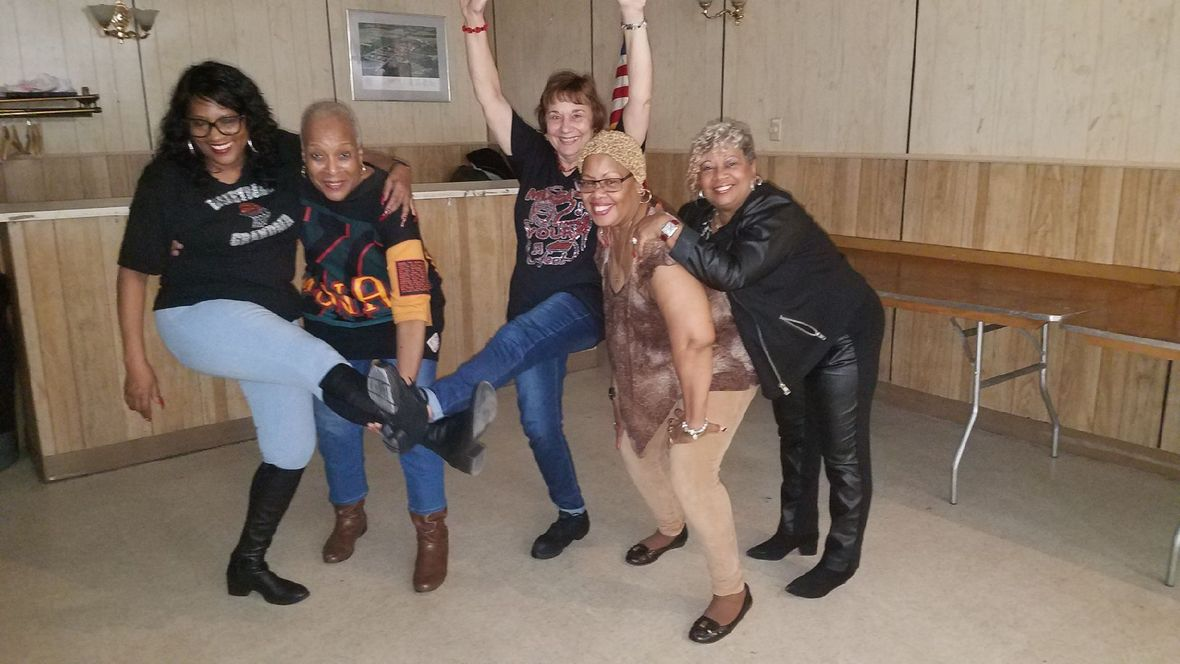Music2YourFeet visits many areas within the community, centers, parks and other events - spreading our love of line dancing. We capture every moment with video and photos. Please check out our photo gallery each month - you may find yourself in them - "Aroundtown"!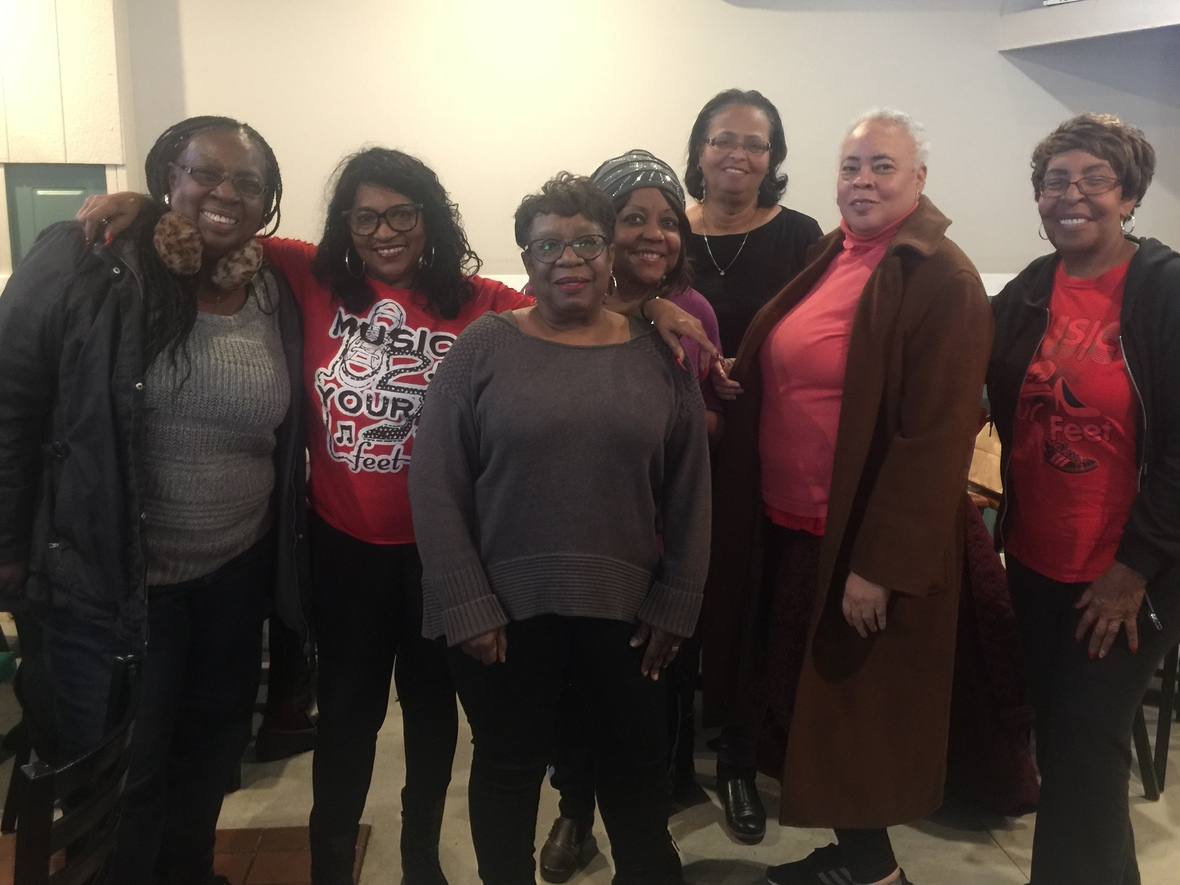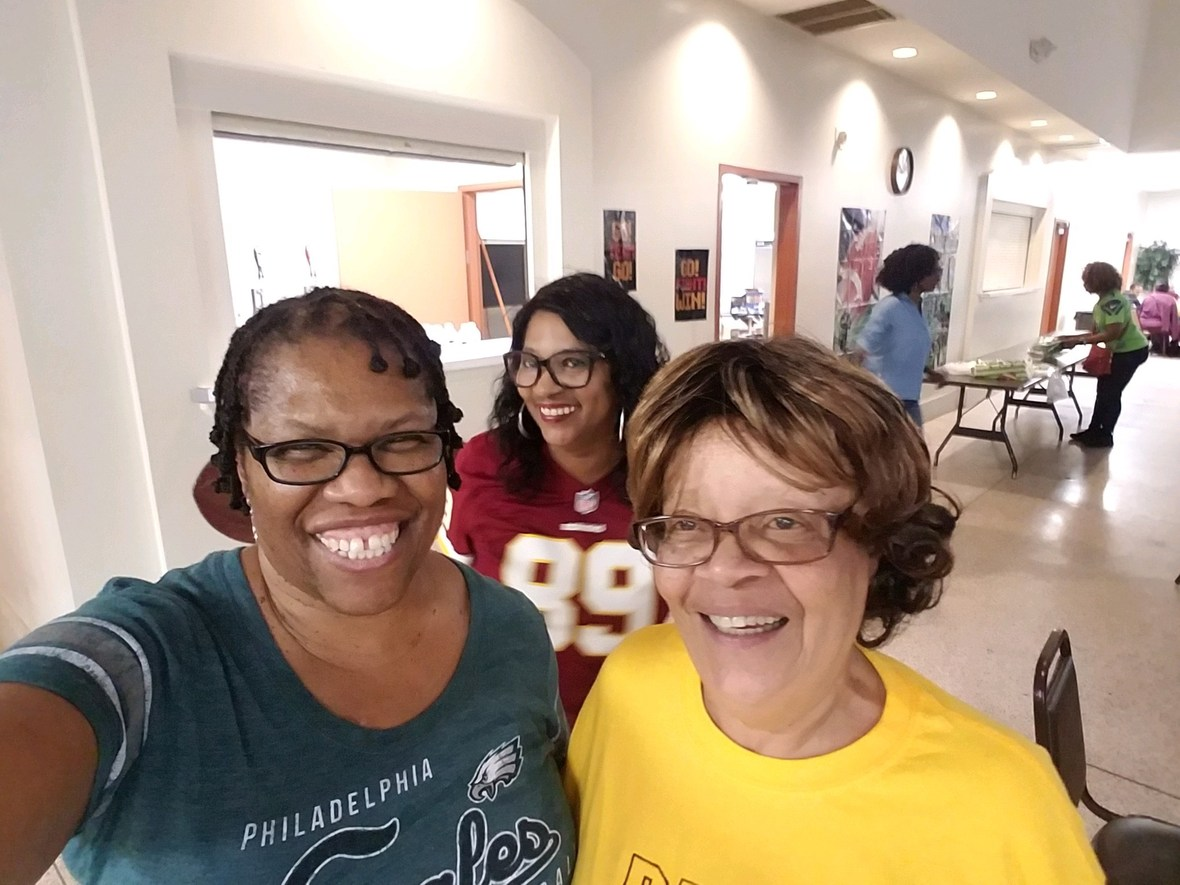table div table+table+table+table+table+table+table+table+table+table+table+table+table+table+table+table+table+table+table+table+table+table+table+table+table+table+table+table+table+table+table+table+table+table+table+table+table+table+table+table+table+table div table{width:100%;padding:0}table div table+table+table+table+table+table+table+table+table+table+table+table+table+table+table+table+table+table+table+table+table+table+table+table+table+table+table+table+table+table+table+table+table+table+table+table+table+table+table+table+table+table div table img{width:96.23%;padding:0;float:none}table div table+table+table+table+table+table+table+table+table+table+table+table+table+table+table+table+table+table+table+table+table+table+table+table+table+table+table+table+table+table+table+table+table+table+table+table+table+table+table+table+table+table div table td{width:100%;padding:0 1.88% 18px}/* styles */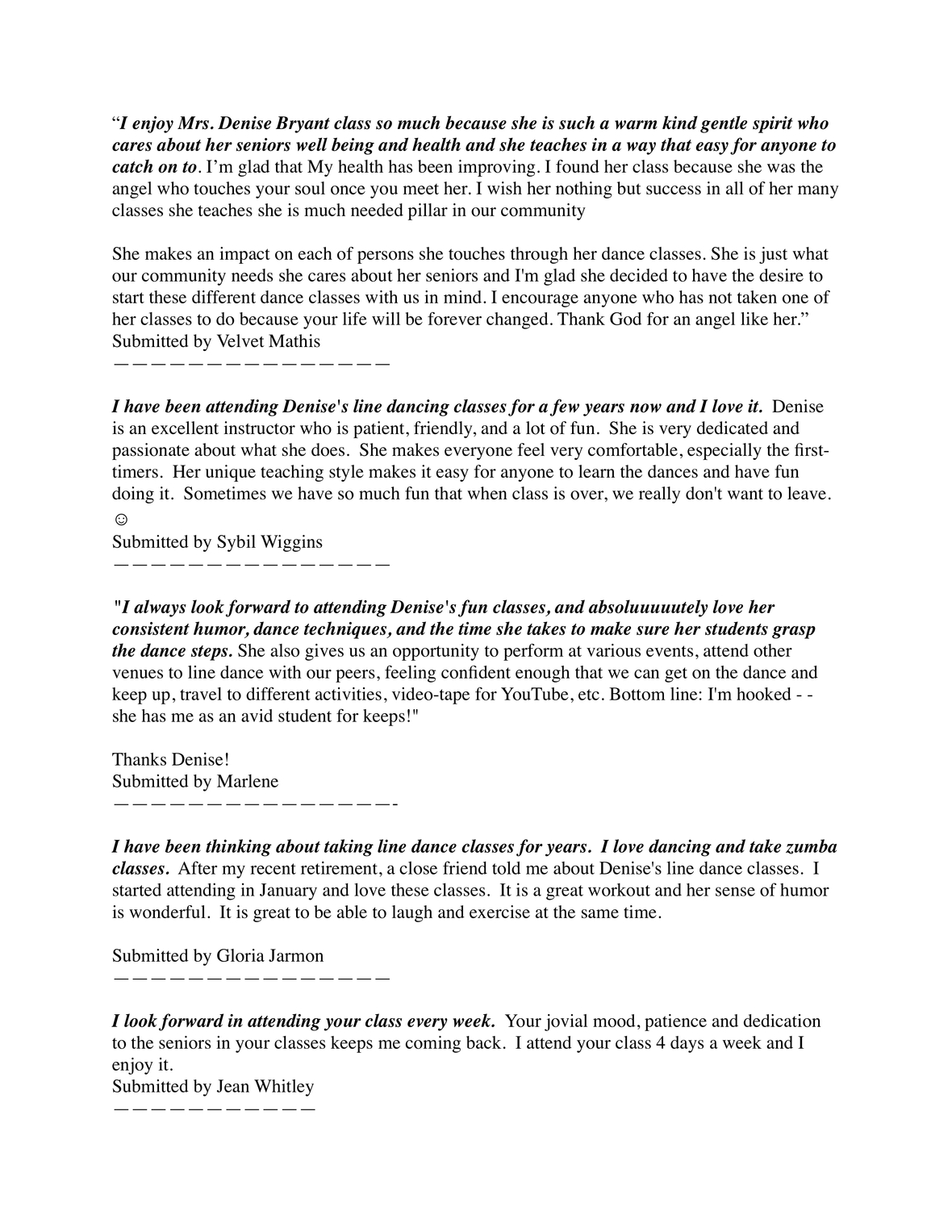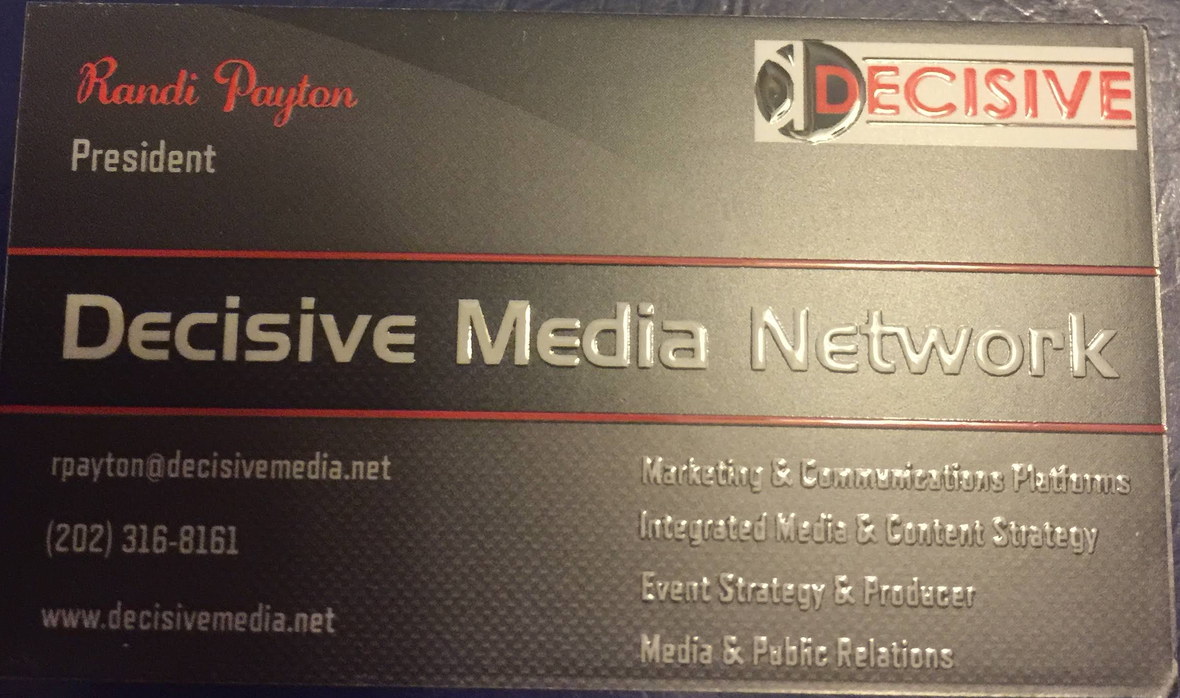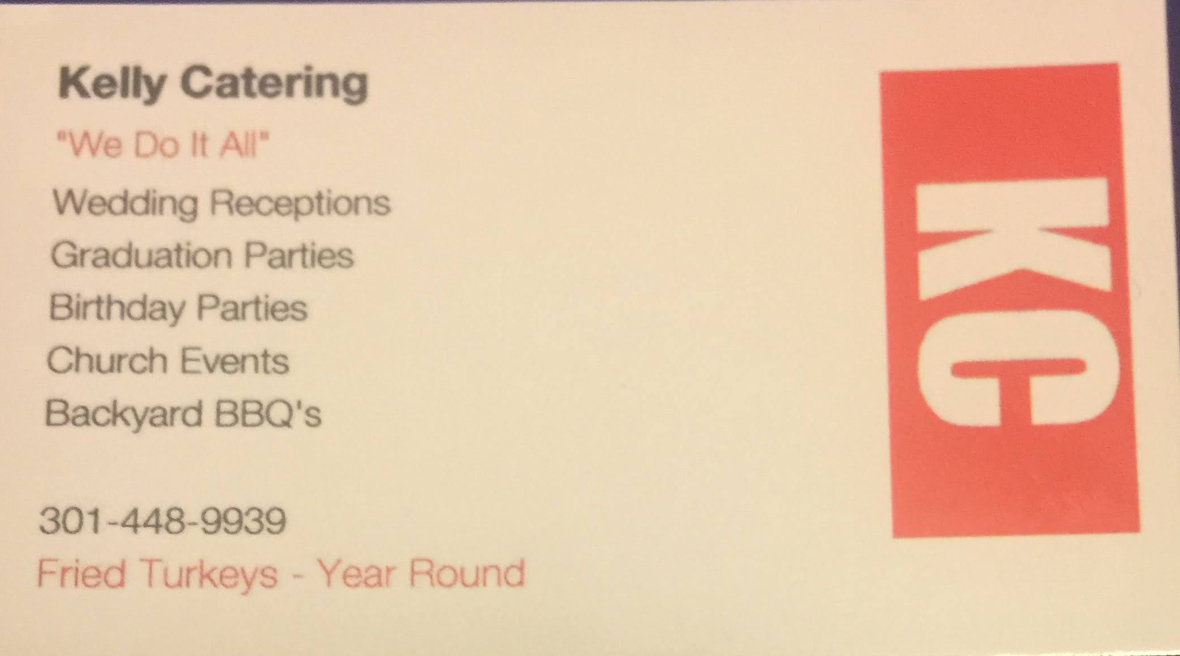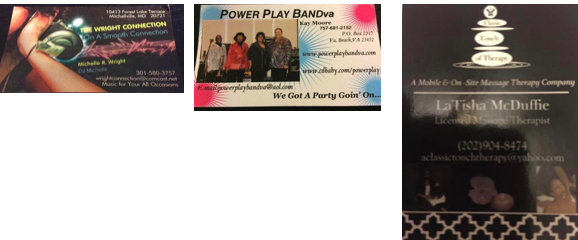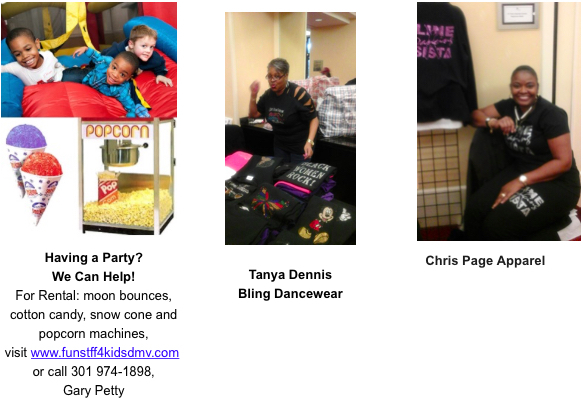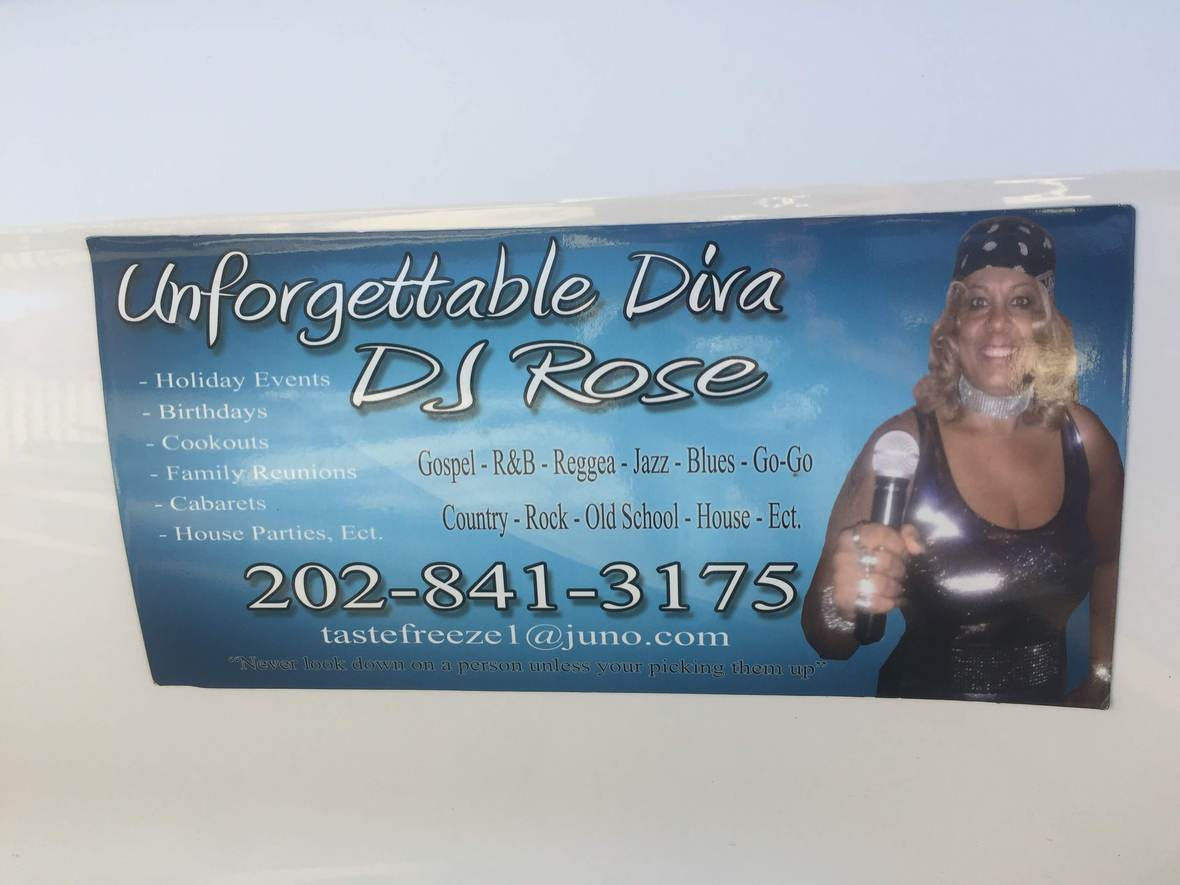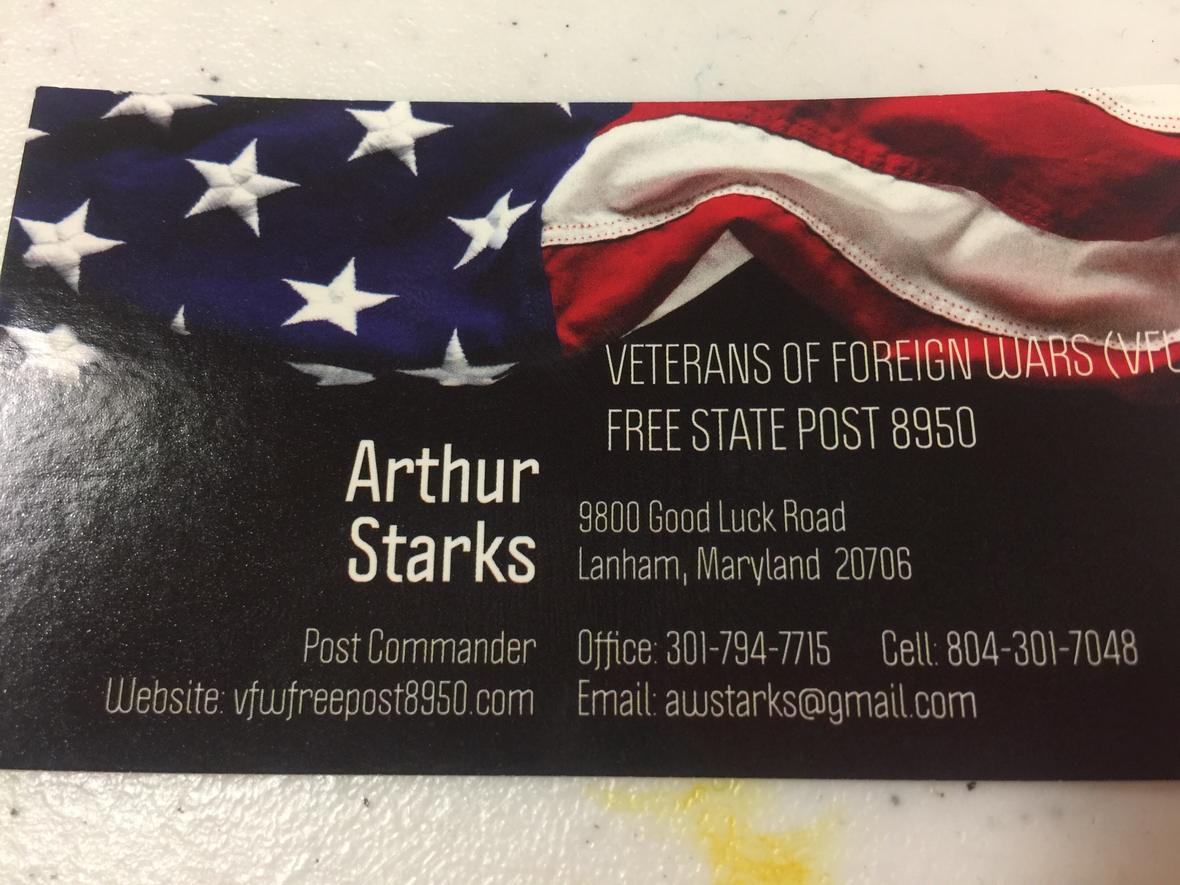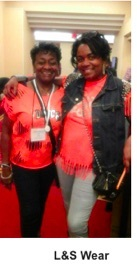# Thank you for your continued support!

 table div table+table+table+table+table+table+table+table+table+table+table+table+table+table+table+table+table+table+table+table+table+table+table+table+table+table+table+table+table+table+table+table+table+table+table+table+table+table+table+table+table+table+table+table+table+table+table+table+table+table+table+table+table+table+table div table{width:100%;padding:0}table div table+table+table+table+table+table+table+table+table+table+table+table+table+table+table+table+table+table+table+table+table+table+table+table+table+table+table+table+table+table+table+table+table+table+table+table+table+table+table+table+table+table+table+table+table+table+table+table+table+table+table+table+table+table+table div table img{width:96.23%;padding:0;float:none}table div table+table+table+table+table+table+table+table+table+table+table+table+table+table+table+table+table+table+table+table+table+table+table+table+table+table+table+table+table+table+table+table+table+table+table+table+table+table+table+table+table+table+table+table+table+table+table+table+table+table+table+table+table+table+table div table td{width:100%;padding:0 1.88% 18px}/* styles */## Music2YourFeet Classes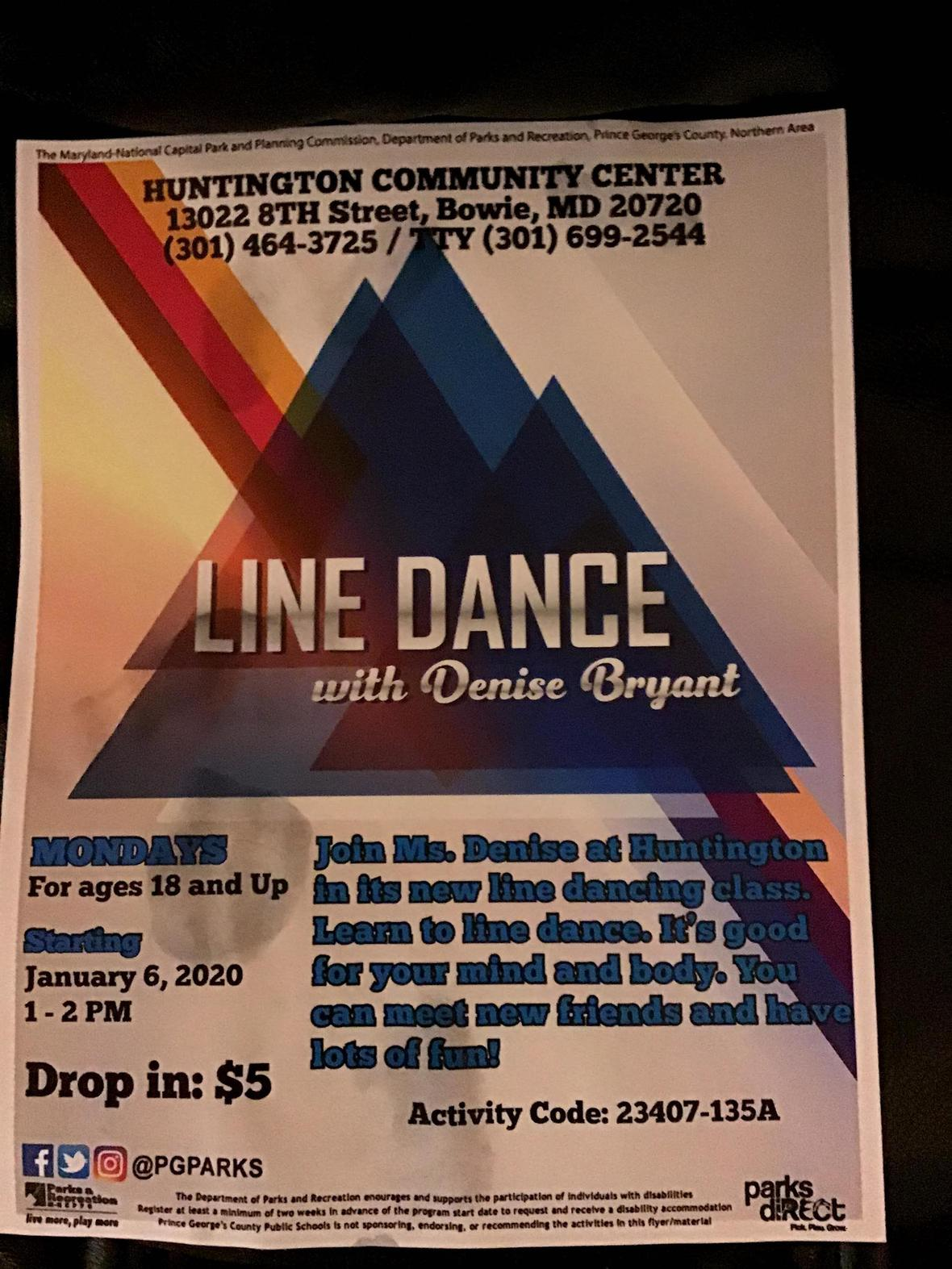Music2yourfeet line dance is at Huntington Community Center/Old Antique Bowie!

Beginners and Seniors, but all are welcome....come out for some fun and exercise, no experience necessary...every Monday at 1:00, see you there.

When: Mondays 1:00-2:00, \$5 drop in
Where: 13022 8th Street, Bowie, Md.

 /* styles */ Monday Lake Arbor Community Center 10100 Lake Arbor Way, Mitchellville, Md. 20721 10:00am-11:30am Calling all Beginners & Seniors, come out for some fun and exercise with Denise Monday Huntington Community Center 13022 8th Street Bowie, MD 1:00pm - 2:00pm \$5 drop in Tuesday Cedar Heights Community Center 1200 Glen Willow Drive Seat Pleasant, MD 9:00am-10:30am \$6, Intermediate Tuesday Glenn Dale Community Center 11901 Glenn Dale Blvd, Glenn Dale, MD 20769 11-12:30pm Beginner \$20 for residents and \$24 for Non-residents. \$5 drop in fee. Beginner Wednesday Good Luck Community Ctr. 6:30-8:00pm 8601 Good Luck Rd, Lanham, Md. 20706 Beginner Friday Ceder Heights Community Center 9am-10:30am 1200 Glen Willow Dr, Capitol Heights, MD 20743 Beginner Friday Senior Day Good Luck Community Center (on-going) 8601 Good Luck Rd Lanham, Md. 11:30am-1:30pm Seniors 60+ FREE, under 60 yrs. Old \$6 Music2YourFeet Private Lessons Available
 table div table+table+table+table+table+table+table+table+table+table+table+table+table+table+table+table+table+table+table+table+table+table+table+table+table+table+table+table+table+table+table+table+table+table+table+table+table+table+table+table+table+table+table+table+table+table+table+table+table+table+table+table+table+table+table+table+table+table+table+table div table{width:100%;padding:0}table div table+table+table+table+table+table+table+table+table+table+table+table+table+table+table+table+table+table+table+table+table+table+table+table+table+table+table+table+table+table+table+table+table+table+table+table+table+table+table+table+table+table+table+table+table+table+table+table+table+table+table+table+table+table+table+table+table+table+table+table div table img{width:96.23%;padding:0;float:none}table div table+table+table+table+table+table+table+table+table+table+table+table+table+table+table+table+table+table+table+table+table+table+table+table+table+table+table+table+table+table+table+table+table+table+table+table+table+table+table+table+table+table+table+table+table+table+table+table+table+table+table+table+table+table+table+table+table+table+table+table div table td{width:100%;padding:0 1.88% 18px}/* styles */## DMV Monthly Dance Socials

 /* styles */ First Sunday of Each Month Sunday Line Dance Social - at Cedar Heights Community Center, 1200 Glen Willow Drive, Seat Pleasant, Md. 3pm - 7pm \$6 for Res & Non-Res. First Friday Line Dance Socials with April Love Jones Southern Regional Technology and Recreation Complex, 6pm-9:30pm includes light refreshments, \$5 resident \$6 non resident First and Third Friday Delight featuring DJ Zone @ VFW Post 7916 (upstairs), 204 Mill Street Occoquan, Va 22125. Time: 7:30 PM to 11:30 PM. Cost: \$10. Open dancing, Cash Bar, Bring your own Food or purchase from the VFW. Follow DJ Zone on Facebook under Vincent Price and join my DJ Zone Status Group for other events and dates. First Saturday of Each Month - Toast it Up Productions - Shut Up & Dance, 1:00-5:00pm, Elk's Lodge, 7350 Temple Hills, Md. BYOF, \$7.00 Third Saturday of Each Month S.O.S. (Seniors On Step) line dance social; 6:00-10:00pm; Cost: Free; BYOFood & beverage (no alcohol permitted) Kingstowne Community Center for Active Adults, 6488 Landsdowne Centre Drive, Alexandria, VA 22315. Every Second Friday, \$5 6p-9p. Learn the latest line dances with Peytrienne! All are welcome, water provided, Soul Line & Hand dance Party, White Oak Community Center, 1700 April Lane Silver Spring, Md. 20904 Every Sunday Elks Lodge of Camp Springs 7350 Temple Hills Rd Temple Hills Md, Hand Dance, Bop, Line Dance & more 6p-10p Fourth Friday of the Month - Style & Rhythm, 4th Friday social ,\$5, Ridgley's Run Community Ctr. 8400 Mission Road, Jessup, Md.
 table div table+table+table+table+table+table+table+table+table+table+table+table+table+table+table+table+table+table+table+table+table+table+table+table+table+table+table+table+table+table+table+table+table+table+table+table+table+table+table+table+table+table+table+table+table+table+table+table+table+table+table+table+table+table+table+table+table+table+table+table+table+table+table div table{width:100%;padding:0}table div table+table+table+table+table+table+table+table+table+table+table+table+table+table+table+table+table+table+table+table+table+table+table+table+table+table+table+table+table+table+table+table+table+table+table+table+table+table+table+table+table+table+table+table+table+table+table+table+table+table+table+table+table+table+table+table+table+table+table+table+table+table+table div table img{width:96.23%;padding:0;float:none}table div table+table+table+table+table+table+table+table+table+table+table+table+table+table+table+table+table+table+table+table+table+table+table+table+table+table+table+table+table+table+table+table+table+table+table+table+table+table+table+table+table+table+table+table+table+table+table+table+table+table+table+table+table+table+table+table+table+table+table+table+table+table+table div table td{width:100%;padding:0 1.88% 18px}/* styles *//* styles */ Denise's "Music2YourFeet" Line Dancing provides you with the opportunity to exercise socialize and have fun - all in one setting. Break the monotony of a long day at work, (evening classes); get mid-day relief to an otherwise stressful day (lunch time classes); Relax after a long work week (weekend classes); or one-on-one personal service (private classes, no matter the day, it will be music2yourfeet Submit your events. Donations not required but appreciated.
 table div table+table+table+table+table+table+table+table+table+table+table+table+table+table+table+table+table+table+table+table+table+table+table+table+table+table+table+table+table+table+table+table+table+table+table+table+table+table+table+table+table+table+table+table+table+table+table+table+table+table+table+table+table+table+table+table+table+table+table+table+table+table+table+table+table+table div table{width:100%;padding:0}table div table+table+table+table+table+table+table+table+table+table+table+table+table+table+table+table+table+table+table+table+table+table+table+table+table+table+table+table+table+table+table+table+table+table+table+table+table+table+table+table+table+table+table+table+table+table+table+table+table+table+table+table+table+table+table+table+table+table+table+table+table+table+table+table+table+table div table img{width:96.23%;padding:0;float:none}table div table+table+table+table+table+table+table+table+table+table+table+table+table+table+table+table+table+table+table+table+table+table+table+table+table+table+table+table+table+table+table+table+table+table+table+table+table+table+table+table+table+table+table+table+table+table+table+table+table+table+table+table+table+table+table+table+table+table+table+table+table+table+table+table+table+table div table td{width:100%;padding:0 1.88% 18px}/* styles */## More Line Dance Classes

 /* styles */ Garland Christopher Tuesday only class 11:00a-12:00pm Palmer Park Community Center 7720 Barlowe Rd, Landover, MD 20785 (301) 773-5665
 table div table+table+table+table+table+table+table+table+table+table+table+table+table+table+table+table+table+table+table+table+table+table+table+table+table+table+table+table+table+table+table+table+table+table+table+table+table+table+table+table+table+table+table+table+table+table+table+table+table+table+table+table+table+table+table+table+table+table+table+table+table+table+table+table+table+table+table+table+table div table{width:100%;padding:0}table div table+table+table+table+table+table+table+table+table+table+table+table+table+table+table+table+table+table+table+table+table+table+table+table+table+table+table+table+table+table+table+table+table+table+table+table+table+table+table+table+table+table+table+table+table+table+table+table+table+table+table+table+table+table+table+table+table+table+table+table+table+table+table+table+table+table+table+table+table div table img{width:96.23%;padding:0;float:none}table div table+table+table+table+table+table+table+table+table+table+table+table+table+table+table+table+table+table+table+table+table+table+table+table+table+table+table+table+table+table+table+table+table+table+table+table+table+table+table+table+table+table+table+table+table+table+table+table+table+table+table+table+table+table+table+table+table+table+table+table+table+table+table+table+table+table+table+table+table div table td{width:100%;padding:0 1.88% 18px}/* styles */## March 2020

▪ Mar 1
♪ DMV'S FIRST SUNDAY LINE DANCE SOCIAL ♪ 2020
3:00 PM- 7:00 PM · Cedar Heights Community Center
▪ Mar 13
An Evening with Shuga Shang with Special Guest Robert T
8 PM · The Carlyle Club
 ▪ Mar 1 ♪ DMV'S FIRST SUNDAY LINE DANCE SOCIAL ♪ 2020 3:00 PM- 7:00 PM · Cedar Heights Community Center
 ▪ Mar 13 An Evening with Shuga Shang with Special Guest Robert T 8 PM · The Carlyle Club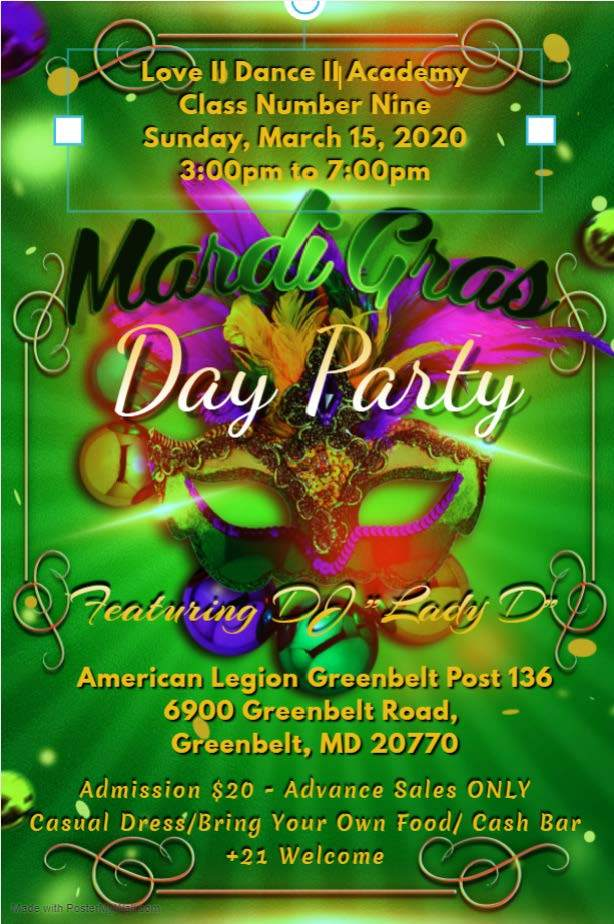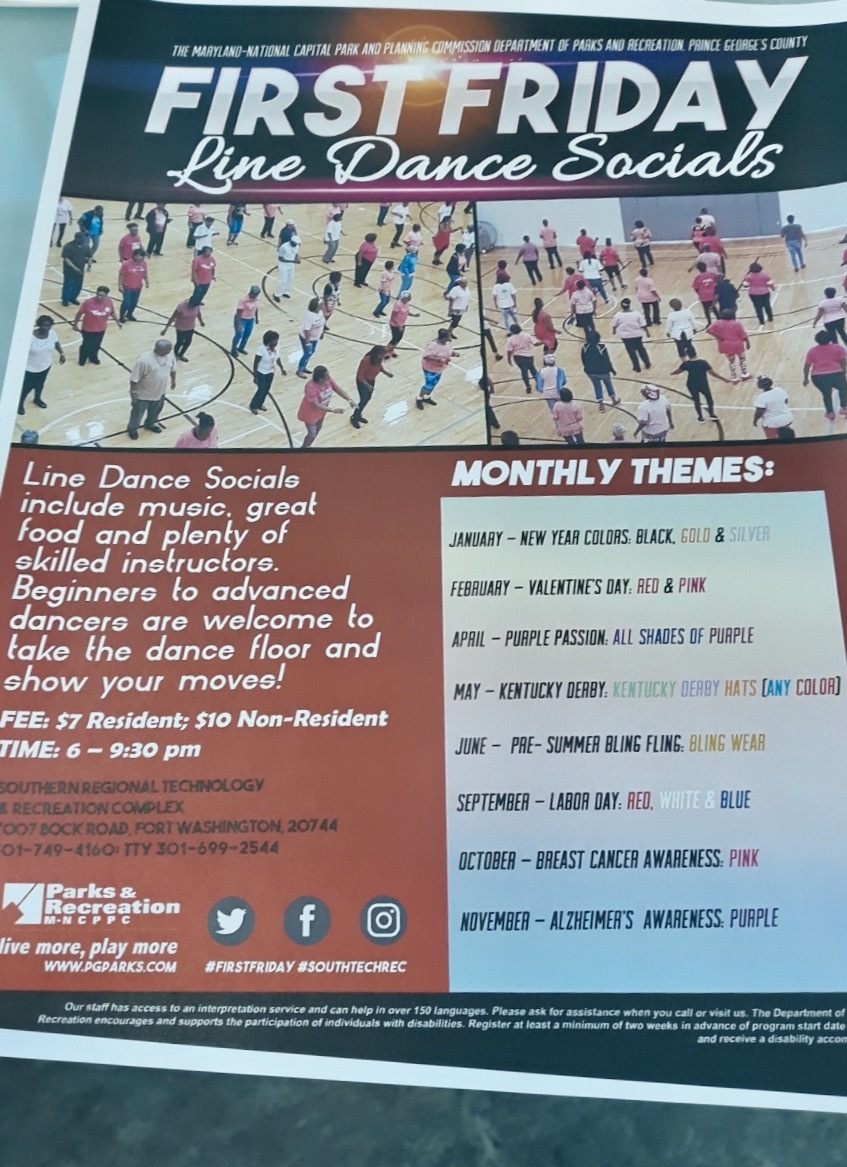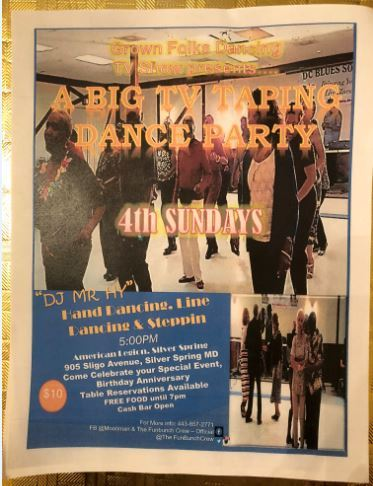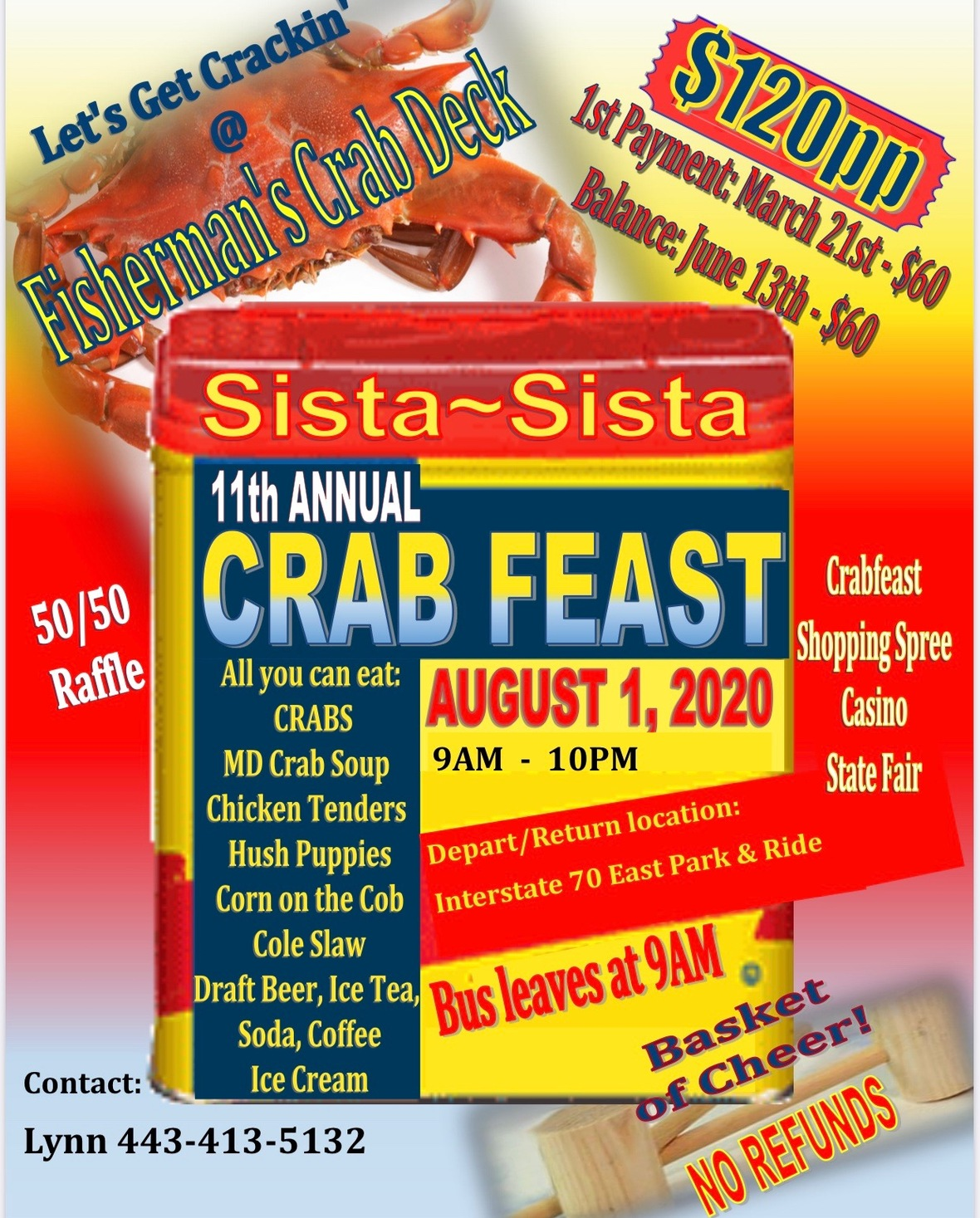table div table+table+table+table+table+table+table+table+table+table+table+table+table+table+table+table+table+table+table+table+table+table+table+table+table+table+table+table+table+table+table+table+table+table+table+table+table+table+table+table+table+table+table+table+table+table+table+table+table+table+table+table+table+table+table+table+table+table+table+table+table+table+table+table+table+table+table+table+table+table+table+table+table+table+table+table+table+table div table{width:100%;padding:0}table div table+table+table+table+table+table+table+table+table+table+table+table+table+table+table+table+table+table+table+table+table+table+table+table+table+table+table+table+table+table+table+table+table+table+table+table+table+table+table+table+table+table+table+table+table+table+table+table+table+table+table+table+table+table+table+table+table+table+table+table+table+table+table+table+table+table+table+table+table+table+table+table+table+table+table+table+table+table div table img{width:96.23%;padding:0;float:none}table div table+table+table+table+table+table+table+table+table+table+table+table+table+table+table+table+table+table+table+table+table+table+table+table+table+table+table+table+table+table+table+table+table+table+table+table+table+table+table+table+table+table+table+table+table+table+table+table+table+table+table+table+table+table+table+table+table+table+table+table+table+table+table+table+table+table+table+table+table+table+table+table+table+table+table+table+table+table div table td{width:100%;padding:0 1.88% 18px}/* styles */